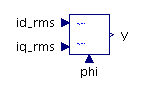Flow Rate Sensor - MapleSim Help

Flow Rate Sensor

Sense flow rate through portsDescription The Flow Rate Sensor component generates an output signal proportional to the flow rate through its ports.Equations $y=\mathrm{toUnit}q$ $q={q}_{A}=-{q}_{B}$ $p={p}_{A}-{p}_{B}=0$Variables

 Name Units Description Modelica ID $p$ $\mathrm{Pa}$ Pressure across component p $q$ $\frac{{m}^{3}}{s}$ Flow rate through component qConnections

 Name Description Modelica ID $\mathrm{portA}$ Upstream hydraulic port portA $\mathrm{portB}$ Downstream hydraulic port portB $y$ Real output; flow rate in selected units yParameters

 Name Default Units Description Modelica ID $\mathrm{toUnit}$ $\frac{{m}^{3}}{s}$ Selected flow-rate unit toUnit